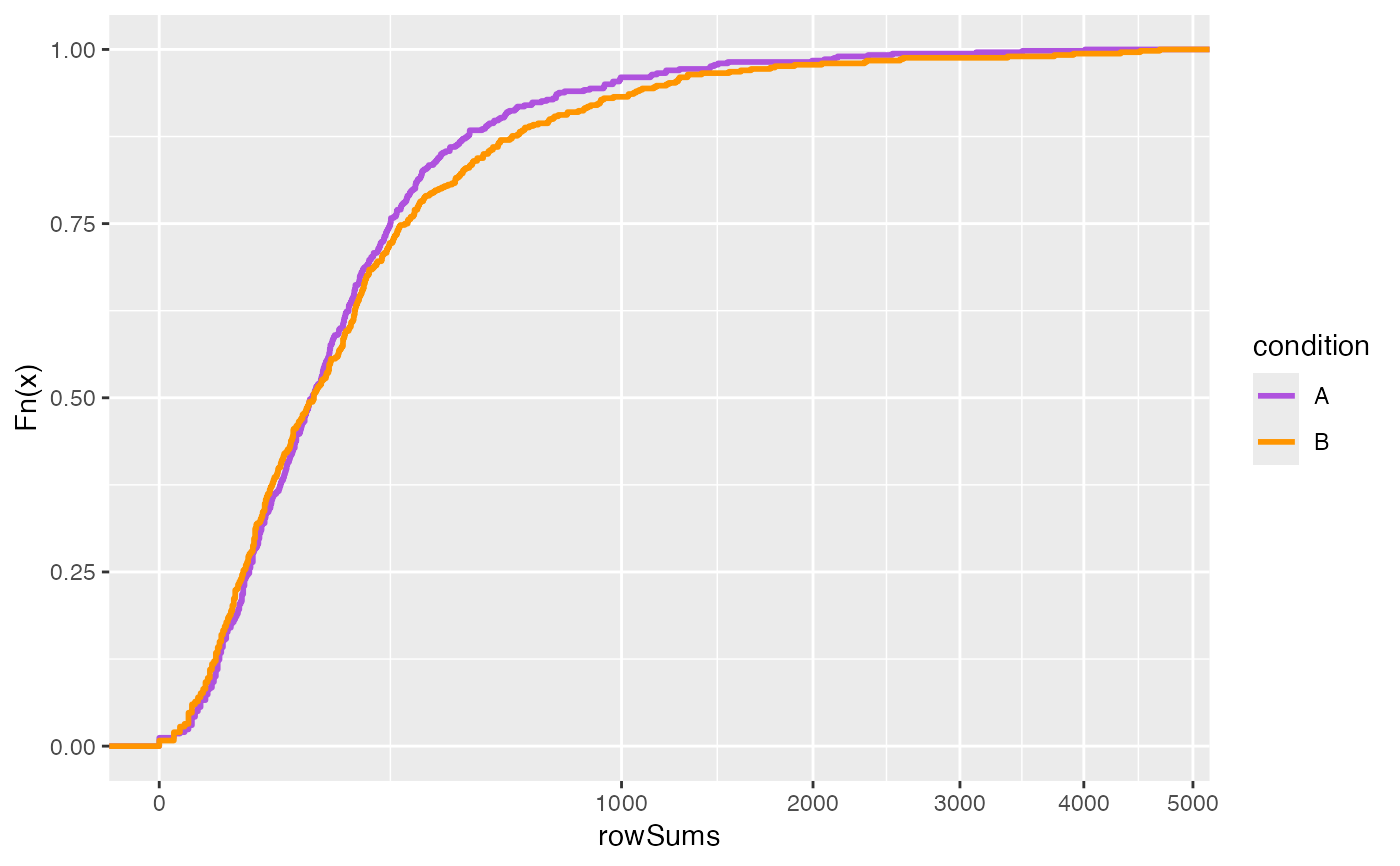Visualize row or column sums using empirical cumulative distribution function (ECDF) plot.

## Usage

plotSums(object, ...)

# S4 method for SummarizedExperiment
plotSums(
object,
MARGIN,
assay = 1L,
interestingGroups = NULL,
labels = list(title = NULL, subtitle = NULL)
)

## Arguments

object

Object.

MARGIN

integer(1-2). Dimension where the function will be applied. For a two-dimensional matrix: 1 indicates rows; 2 indicates columns; c(1, 2) indicates rows and columns.

assay

vector(1). Assay name or index position.

interestingGroups

character. Groups of interest to use for visualization. Corresponds to factors describing the columns of the object.

labels

list. ggplot2 labels. See ggplot2::labs() for details.

...

## Value

ggplot.

## Note

Updated 2023-08-10.

## Examples

data(
RangedSummarizedExperiment,
package = "AcidTest"
)

## SummarizedExperiment ====
object <- RangedSummarizedExperiment
plotSums(object, MARGIN = 1L)plotSums(object, MARGIN = 2L)